search for books and compare prices
curves algebraic matches 62 work(s)
at beginning | displaying 1 to 25 | next 25 >
show results in order: alphabetically | oldest to newest | newest to oldest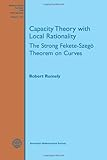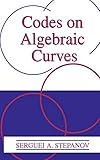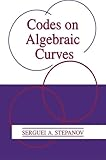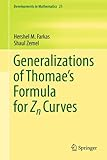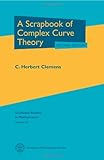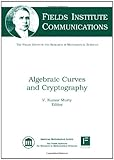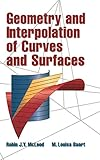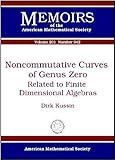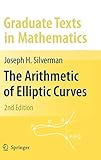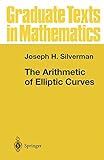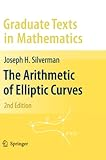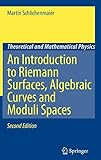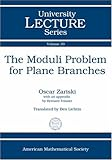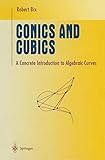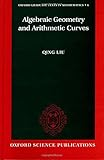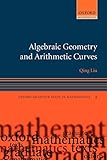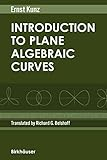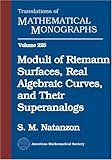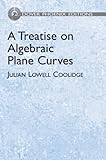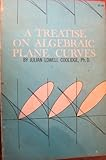Product Description: The Dynamical Mordell-Lang Conjecture is an analogue of the classical Mordell-Lang conjecture in the context of arithmetic dynamics. It predicts the behavior of the orbit of a point \$x\$ under the action of an endomorphism \$f\$ of a quasiprojective complex variety \$X\$...read more

Hardcover:

9781470424084 | Amer Mathematical Society, April 20, 2016, cover price \$110.00 | About this edition: The Dynamical Mordell-Lang Conjecture is an analogue of the classical Mordell-Lang conjecture in the context of arithmetic dynamics.

Product Description: In May of 1868, Elizabeth Bingham Young and her new husband began a long journey from Hamilton, Ontario, to the Methodist mission of Rossville. For the next eight years, she lived in two mission houses, Norway House and then Berens River...read more

Paperback:

9781771990035 | Athabasca Univ, March 19, 2015, cover price \$32.95 | About this edition: In May of 1868, Elizabeth Bingham Young and her new husband began a long journey from Hamilton, Ontario, to the Methodist mission of Rossville.
9780387514109, titled "Capacity Theory on Algebraic Curves" | Springer Verlag, October 1, 1989, cover price \$56.95 | also contains Capacity Theory on Algebraic Curves

Product Description: This book is devoted to the proof of a deep theorem in arithmetic geometry, the Fekete-SzegÃ¶ theorem with local rationality conditions. The prototype for the theorem is Raphael Robinson's theorem on totally real algebraic integers in an interval, which says that if is a real interval of length greater than 4, then it contains infinitely many Galois orbits of algebraic integers, while if its length is less than 4, it contains only finitely many...read more

Hardcover:

9781470409807 | Amer Mathematical Society, January 22, 2014, cover price \$119.00 | About this edition: This book is devoted to the proof of a deep theorem in arithmetic geometry, the Fekete-SzegÃ¶ theorem with local rationality conditions.

Product Description: This is a self-contained introduction to algebraic curves over finite fields and geometric Goppa codes. There are four main divisions in the book. The first is a brief exposition of basic concepts and facts of the theory of error-correcting codes (Part I)...read more

Hardcover:

9780306461446 | Plenum Pub Corp, July 31, 1999, cover price \$279.00

Paperback:

9781461371670 | Springer Verlag, October 21, 2012, cover price \$279.00 | About this edition: This is a self-contained introduction to algebraic curves over finite fields and geometric Goppa codes.

Hardcover:

9781420079463 | Chapman & Hall, June 13, 2013, cover price \$83.95

Product Description: Previous publications on the generalization of the Thomae formulae to Zn curves have emphasized the theory's implications in mathematical physics and depended heavily on applied mathematical techniques. This book redevelops these previous results demonstrating how they can be derived directly from the basic properties of theta functions as functions on compact Riemann surfaces...read more

Hardcover:

9781441978462 | Springer Verlag, November 6, 2010, cover price \$189.00 | About this edition: Previous publications on the generalization of the Thomae formulae to Zn curves have emphasized the theory's implications in mathematical physics and depended heavily on applied mathematical techniques.

Paperback:

9781461427582 | Springer Verlag, January 2, 2013, cover price \$189.00 | About this edition: Previous publications on the generalization of the Thomae formulae to Zn curves have emphasized the theory's implications in mathematical physics and depended heavily on applied mathematical techniques.

Product Description: This is a book of "impressions" of a journey through the theory of comÂ­ plex algebraic curves. It is neither self-contained, balanced, nor particularly tightly organized. As with any notebook made on a journey, what appears is that which strikes the writer's fancy...read more

Hardcover:

9780821833070, titled "A Scrapbook of Complex Curve Theory" | 2 edition (Amer Mathematical Society, November 1, 2002), cover price \$48.00 | About this edition: This fine book by Herb Clemens quickly became a favorite of many algebraic geometers when it was first published in 1980.
9780306405365 | Plenum Pub Corp, June 1, 1981, cover price \$99.00 | About this edition: This is a book of "impressions" of a journey through the theory of comÂ­ plex algebraic curves.

Paperback:

9781468470024, titled "A Scrapbook of Complex Curve Theory" | Reprint edition (Springer Verlag, April 2, 2012), cover price \$99.00 | About this edition: This is a book of "impressions" of a journey through the theory of comÂ­ plex algebraic curves.

Product Description: It is by now a well-known paradigm that public-key cryptosystems can be built using finite Abelian groups and that algebraic geometry provides a supply of such groups through Abelian varieties over finite fields. Of special interest are the Abelian varieties that are Jacobians of algebraic curves...read more
By V. Kumar Murty (editor)

Hardcover:

9780821843116 | Amer Mathematical Society, November 24, 2010, cover price \$83.00 | About this edition: It is by now a well-known paradigm that public-key cryptosystems can be built using finite Abelian groups and that algebraic geometry provides a supply of such groups through Abelian varieties over finite fields.

Product Description: This text takes a practical, step-by-step approach to algebraic curves and surface interpolation motivated by the understanding of the many applications in engineering analysis, approximation, and curve plotting problems. Because of its usefulness for computing, the algebraic approach is the main theme, but a brief discussion of the synthetic approach is also presented as a way of gaining additional insight before proceeding with the algebraic manipulation...read more (view table of contents, read Amazon.com's description)

Hardcover:

9780521321532 | Cambridge Univ Pr, August 1, 1998, cover price \$155.00 | About this edition: This text takes a practical, step-by-step approach to algebraic curves and surface interpolation motivated by the understanding of the many applications in engineering analysis, approximation, and curve plotting problems.

Product Description: In these notes the author investigates noncommutative smooth projective curves of genus zero, also called exceptional curves. As a main result he shows that each such curve X admits, up to some weighting, a projective coordinate algebra which is a not necessarily commutative graded factorial domain R in the sense of Chatters and Jordan...read more

Paperback:

9780821844007 | Amer Mathematical Society, September 11, 2009, cover price \$71.00 | About this edition: In these notes the author investigates noncommutative smooth projective curves of genus zero, also called exceptional curves.

The theory of elliptic curves is distinguished by its long history and by the diversity of the methods that have been used in its study. This book treats the arithmetic theory of elliptic curves in its modern formulation, through the use of basic algebraic number theory and algebraic geometry. The book begins with a brief discussion of the necessary algebro-geometric results, and proceeds with an exposition of the geometry of elliptic curves, the formal group of an elliptic curve, elliptic curves over finite fields, the complex numbers, local fields, and global fields. The last two chapters deal with integral and rational points, including Siegel's theorem and explicit computations for the curve Y^2 = X^3 + DX. The book contains three appendices: Elliptic Curves in Characteristics 2 and 3, Group Cohomology, and a third appendix giving an overview of more advanced topics.

Hardcover:

9780387094939 | 2 edition (Springer Verlag, June 12, 2009), cover price \$59.95
9780387962030 | Springer Verlag, January 1, 1986, cover price \$69.95 | About this edition: The theory of elliptic curves is distinguished by its long history and by the diversity of the methods that have been used in its study.

Paperback:

9781441918581 | Springer Verlag, May 29, 2009, cover price \$59.95

Hardcover:

9780691096797 | Princeton Univ Pr, March 3, 2008, cover price \$132.00

Paperback:

Product Description: This self-contained 2007 textbook presents an exposition of the well-known classical two-dimensional geometries, such as Euclidean, spherical, hyperbolic, and the locally Euclidean torus, and introduces the basic concepts of Euler numbers for topological triangulations, and Riemannian metrics...read more

Hardcover:

9780521886291 | 1 edition (Cambridge Univ Pr, January 21, 2008), cover price \$170.00 | About this edition: This self-contained 2007 textbook presents an exposition of the well-known classical two-dimensional geometries, such as Euclidean, spherical, hyperbolic, and the locally Euclidean torus, and introduces the basic concepts of Euler numbers for topological triangulations, and Riemannian metrics.

Paperback:

9780521713900 | 1 edition (Cambridge Univ Pr, January 31, 2008), cover price \$59.99 | About this edition: This self-contained 2007 textbook presents an exposition of the well-known classical two-dimensional geometries, such as Euclidean, spherical, hyperbolic, and the locally Euclidean torus, and introduces the basic concepts of Euler numbers for topological triangulations, and Riemannian metrics.

Paperback:

9780821843239 | Amer Mathematical Society, December 13, 2007, cover price \$29.00

This book gives an introduction to modern geometry. Starting from an elementary level, the author develops deep geometrical concepts that play an important role in contemporary theoretical physics, presenting various techniques and viewpoints along the way. This second edition contains two additional, more advanced geometric techniques: the modern language and modern view of Algebraic Geometry and Mirror Symmetry.

Hardcover:

9783540711742 | 2 edition (Springer Verlag, October 4, 2007), cover price \$89.95
9780387501246 | Springer Verlag, March 1, 1989, cover price \$34.95 | About this edition: This book gives an introduction to modern geometry.

Paperback:

9783642090271 | 2 edition (Springer Verlag, October 4, 2007), cover price \$89.95 | About this edition: This book gives an introduction to modern geometry.

Product Description: Moduli problems in algebraic geometry date back to Riemann's famous count of the \$3g-3\$ parameters needed to determine a curve of genus \$g\$. In this book, Zariski studies the moduli space of curves of the same equisingularity class...read more
By Ben Lichtin (trans), Bernard Teissier (contributor) and Oscar Zariski

Paperback:

9780821829837 | Amer Mathematical Society, January 1, 2007, cover price \$38.00 | About this edition: Moduli problems in algebraic geometry date back to Riemann's famous count of the \$3g-3\$ parameters needed to determine a curve of genus \$g\$.

Product Description: Here is a deformation theory for degenerations of complex curves; specifically, discussing deformations which induce splitting of the singular fiber of a degeneration. The author constructs a deformation of the degeneration in such a way that a subdivisor is "barked," or peeled off from the singular fiber...read more

Paperback:

9783540333630 | Springer Verlag, September 15, 2006, cover price \$109.00 | About this edition: Here is a deformation theory for degenerations of complex curves; specifically, discussing deformations which induce splitting of the singular fiber of a degeneration.

Product Description: Conics and Cubics offers an accessible and well illustrated introduction to algebraic curves. By classifying irreducible cubics over the real numbers and proving that their points form Abelian groups, the book gives readers easy access to the study of elliptic curves...read more

Hardcover:

9780387318028 | 2 edition (Springer Verlag, August 30, 2006), cover price \$64.95 | About this edition: Conics and Cubics offers an accessible and well illustrated introduction to algebraic curves.
9780387984018 | Springer Verlag, July 1, 1998, cover price \$79.95 | About this edition: An accessible introduction to algebraic curves which focuses on curves of degree at most three keeps results tangible and proofs transparent.

Paperback:

9781441921789 | 2 edition (Springer Verlag, August 30, 2006), cover price \$64.95 | About this edition: Conics and Cubics offers an accessible and well illustrated introduction to algebraic curves.

This book is a general introduction to the theory of schemes, followed by applications to arithmetic surfaces and to the theory of reduction of algebraic curves. The first part introduces basic objects such as schemes, morphisms, base change, local properties (normality, regularity, Zariski's Main Theorem). This is followed by the more global aspect: coherent sheaves and a finiteness theorem for their cohomology groups. Then follows a chapter on sheaves of differentials, dualizing sheaves, and grothendieck's duality theory. The first part ends with the theorem of Riemann-Roch and its application to the study of smooth projective curves over a field. Singular curves are treated through a detailed study of the Picard group. The second part starts with blowing-ups and desingularization (embedded or not) of fibered surfaces over a Dedekind ring that leads on to intersection theory on arithmetic surfaces. Castelnuovo's criterion is proved and also the existence of the minimal regular model. This leads to the study of reduction of algebraic curves. The case of elliptic curves is studied in detail. The book concludes with the fundamental theorem of stable reduction of Deligne-Mumford. The book is essentially self-contained, including the necessary material on commutative algebra. The prerequisites are therefore few, and the book should suit a graduate student. It contains many examples and nearly 600 exercises.

Hardcover:

9780198502845 | Oxford Univ Pr on Demand, July 18, 2002, cover price \$150.00 | About this edition: This book is a general introduction to the theory of schemes, followed by applications to arithmetic surfaces and to the theory of reduction of algebraic curves.

Paperback:

9780199202492 | Oxford Univ Pr, August 24, 2006, cover price \$85.00

Vertex algebras were first introduced as a tool used in the description of the algebraic structure of certain quantum field theories. It became increasingly important that vertex algebras are useful not only in the representation theory of infinite-dimensional Lie algebras, where they are by now ubiquitous, but also in other fields, such as algebraic geometry, theory of finite groups, modular functions, and topology. This book is an introduction to the theory of vertex algebras with a particular emphasis on the relationship between vertex algebras and the geometry of moduli spaces of algebraic curves. The authors make the first steps toward reformulating the theory of vertex algebras in a way that is suitable for algebraic-geometric applications. The notion of a vertex algebra is introduced in the book in a co-ordinate independent way, allowing the authors to give global geometric meaning to vertex operators on arbitrary smooth algebraic curves, possibly equipped with some additional data. To each vertex algebra and a smooth curve, they attach an invariant called the space of conformal blocks. When the complex structure of the curve and other geometric data are varied, these spaces combine into a sheaf on the relevant moduli space. From this perspective, vertex algebras appear as the algebraic objects that encode the geometric structure of various moduli spaces associated with algebraic curves. Numerous examples and applications of vertex algebras are included, such as the Wakimoto realization of affine Kac-Moody algebras, integral solutions of the Knizhnik-Zamolodchikov equations, classical and quantum Drinfeld-Sokolov reductions, and the \$W\$-algebras. The authors also establish a connection between vertex algebras and chiral algebras, introduced by A. Beilinson and V. Drinfeld.

Hardcover:

9780821828946 | Amer Mathematical Society, August 1, 2001, cover price \$55.00 | About this edition: Vertex algebras were first introduced as a tool used in the description of the algebraic structure of certain quantum field theories.

Paperback:

9780821836743 | 2 edition (Amer Mathematical Society, September 1, 2004), cover price \$80.00

Product Description: The space of all Riemann surfaces (the so-called moduli space) plays an important role in algebraic geometry and its applications to quantum field theory. The present book is devoted to the study of topological properties of this space and of similar moduli spaces, such as the space of real algebraic curves, the space of mappings, and also superanalogs of all these spaces...read more

Hardcover:

9780821835944 | Amer Mathematical Society, September 1, 2004, cover price \$87.00 | About this edition: The space of all Riemann surfaces (the so-called moduli space) plays an important role in algebraic geometry and its applications to quantum field theory.

Product Description: Students and teachers will welcome the return of this unabridged reprint of one of the first English-language texts to offer full coverage of algebraic plane curves. It offers advanced students a detailed, thorough introduction and background to the theory of algebraic plane curves and their relations to various fields of geometry and analysis...read more

Hardcover:

9780486495767, titled "A Treatise on Algebraic Plane Curves" | Dover Pubns, April 28, 2004, cover price \$75.00 | About this edition: Students and teachers will welcome the return of this unabridged reprint of one of the first English-language texts to offer full coverage of algebraic plane curves.

Paperback:

9780486605432 | Dover Pubns, June 1, 1959, cover price \$5.00 | About this edition: A thorough introduction to the theory of algebraic plane curves and their relations to various fields of geometry and analysis.

at beginning | displaying 1 to 25 | next 25 >